## Minimal Swing Container Transfer Problem

The third example represents the problem of transferring containers, driven by a hoist motor and a trolley drive motor, from a ship to a cargo truck. The goal is to minimize the swing during and at the end of the transfer. The mathematical optimal control problem is described by (1)-(13). The problem is nonlinear with six state variables and two controllers subject to multiple final and bound constraints.

Minimize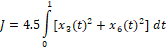(1)

subject to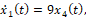(2)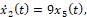(3)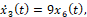(4)(5)(6)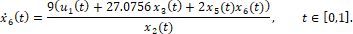(7)

Initial conditions:(8)

Final conditions: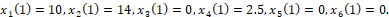(9)

Bounds: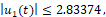(10)(11)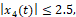(12)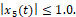(13)

Following the same procedure as that in the previous examples, we define the model for the IVP (2)-(8) using third-order parametrized polynomial control functions as shown in Table 1. Initial values and guesses are assigned to the state variables and unknown parametrization coefficients as shown in the table.

 A B C D 1 ODE variables 2 t 0 3 x_1 0 4 x_2 22 5 x_3 0 6 x_4 0 7 x_5 -1 8 x_6 0 9 Parametrized controls formulas 10 c_0 1 d_0 1 11 c_1 1 d_1 1 12 c_2 -5 d_2 -5 13 c_3 -5 d_3 -5 14 u_1 =c_0+c_1*t+c_2*t^2+c_3*t^3 15 u_2 =d_0+d_1*t+d_2*t^2+d_3*t^3 16 ODE rhs equations 17 x1dot =9*x_4 18 x2dot =9*x_5 19 x3dot =9*x_6 20 x4dot =9*(u_1+x_3) 21 x5dot =9*u_2 22 x6dot =9*(u_1+27.0756*x_3+2*x_5*x_6)/x_2

Table 2 shows a partial listing of the initial solution obtained by evaluating the array formula =IVSOLVE(B17:B22, B2:B8, {0,1}) in array F2:L103, along with the generated control columns for u_1, u_2, and the integrand expression using the corresponding formulas listed in Table 3. The initial system trajectories are plotted in Figure 1.

Next, we defined the objective formula, S3, corresponding to the cost index (17) as shown in Table 3, in which the data integrator QUADXY was used to integrate the generated integrand expression values. The objective formula evaluated to an initial value of 24,229.22793. Table 3 also lists a number of aid formulas which compute the minimum and maximum values for the state variables x_4 and x_5 and the generated control columns. These aid formulas were used to define the bound constraints for the NLP Solver.

 IVP Solution u_1 u_2 Integrand Objective Constraints F G H I J K L N O P R S 1 2 t x_1 x_2 x_3 x_4 x_5 x_6 3 0 0 22 0 0 -1 0 1 1 0 24229.22793 4 0.01 0.004063 21.91406 0.000185 0.09044 -0.90957 0.00411 1.009495 1.009495 1.69E-05 5 0.02 0.016305 21.8363 0.000742 0.181723 -0.81832 0.008285 1.01796 1.01796 6.92E-05 6 0.03 0.036797 21.76679 0.001679 0.273786 -0.72636 0.012569 1.025365 1.025365 0.000161 max(u1) 1.045855 101 0.98 230.6731 15.34798 189.5472 205.5287 -12.3527 147.8948 -7.52796 -7.52796 57801.01 102 0.99 249.9267 14.20542 203.2503 222.511 -13.0407 156.6717 -7.762 -7.762 65856.72 103 1 270.7634 13 217.7568 240.7409 -13.75 165.7417 -8 -8 74888.35
Table 3: formulas used to generate values shown in Table 2
PurposeCellFormula
Initial value problem solutionF2:L103=IVSOLVE(B17:B22, B2:B8, {0,1})
AutoFill formula for u_1 control valuesN3=c_0+c_1*F3+c_2*F3^2+c_3*F3^3
AutoFill formula for u_2 control valuesO3=d_0+d_1*F3+d_2*F3^2+d_3*F3^3
AutoFill formula for integrand valuesP3=I3^2+L3^2
Objective FormulaS3=4.5*QUADXY(F3:F103, P3:P103)
u_1 column max valueS6=MAXA(N3:N103)
u_1 column min valueS7=MINA(N3:N103)
u_2 column max valueS8=MAXA(O3:O103)
u_2 column min valueS9=MINA(O3:O103)
x_4 column max valueS10=MAXA(J3:J103)
x_4 column min valueS11=MINA(J3:J103)
x_5 column max valueS12=MAXA(K3:K103)
x_5 column min valueS13=MINA(K3:K103)

### Results and Discussion

We configured Excel Solver to minimize the objective formula S3 by varying the controls' coefficients B10:B14 and D10:D14 subject to the constraints listed in Table 4. The Solver spun for a few seconds, then reported that it did not find a feasible solution when, in fact, it already had, judging by the best-found solution results shown partially in Figure 2 and plotted in Figure 3. The solution indicates that all constraints were satisfied within a reasonable tolerance of 1x10-5, except for x_6(1), which was satisfied within a tolerance of 1x10-3. This is verified by the feasibility report generated by the Solver, and shown in Figure 4.

Table 4. constraints added to Excel Solver
FormulaConstraint
G103 = 10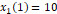H103 = 14I103 = 0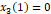J103 = 2.5K103 = 0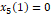L103 = 2.5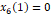S10 ≤ 2.5(12)
S11 ≥ -2.5
S12 ≤ 1(13)
S13 ≥ -1
S6 ≤ 2.83374(10)
S7 ≥ -2.83374
S8 ≤ 0.71265(11)
S9 ≥ -0.80865

#### References

 Elnagar, G.; Kazemi, M.A. Pseudospectral Chebyshev Optimal Control of Constrained Nonlinear Dynamical Systems. Comput. Optim. Appl. 1998, 11, 195-217.

 Ghaddar, C. K. "Novel Spreadsheet Direct Method for Optimal Control Problems." Math. Comput. Appl., 23, 6, 2018.
Available at: http://www.mdpi.com/2297-8747/23/1/6

 Ghaddar, C.K. "Rapid Solution of Optimal Control Problems by a Functional Spreadsheet Paradigm: A Practical Method for the Non-Programmer". Math. Comput. Appl. 2018, 23, 54.
Available at: https://www.mdpi.com/2297-8747/23/4/54

Question or Comment? Email us:
support @ excel-works.com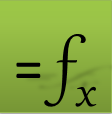ExceLab: Transforming Excel into a Calculus Power House

ExceLab functions and methods are protected by USA Patents 10628634, 10114812, 9892108 and 9286286.